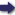(London :  Kegan Paul, Trench, Trübner & Co.,  1910.)

 Tools

## Search this bookPrev Page 364 Next``` 364 ALBERUNTS INDIA. 976/JjT' which is equivalent to 9y6-^rfiJ',j. Vide p. 24 1.53 (Schram). P. 22, 1. 17.—Read 22''i instead of 23"' (Schretm). P. 23. PadamtXset.—Thi^ seems to be an old mistake which has crept into the Arabic manuscripts of the works of Alfazari and Ya'kub. Cf. the author's "Chronology" (English edition), p. 15. P. 27.—The rule given in the first fifteen lines of this page is completely erroneous, and consequently the ex¬ ample calculated after this rule is so too. The right method would be the following :—■" The complete years are multiplied by 12; to the product are added the months which have elapsed of the current year. The sum repre¬ sents the partial solar months. You write down the number in two places ; in the one place you multiply it by ^'^11, i.e. the number which represents the universal adhimasa months. The product you divide by 172,800, i.e. the number which represents the universal solar months. The quotient you get, asfetr as it contains com- qilete months, is added to the number in the second place, and the sum so obtained is multiplied by 30; to the pro¬ duct are added the days which have elapsed of the current month. The sum represents the candrahargana, i.e. the sum of the partial lunar days." These two proceedings would be identical, if we were not to omit fractions ; but as an adhimasa month is only intercalated when it is com¬ plete, we must first determine the number of adhimasa months, and, omitting the fretctions, change them to days; whilst when we multiply beforehand by 30, the fractions of the adhimasa months are also multiplied, which is not correct. This is at once seen in the example which he works out after this rule, and we wonder that Albe¬ runi himself did not see' it. He is calculating the ahar- ganas for the beginning of a year, consequently also for the beginning of a month, and, notwithstanding, he is not at all surprised to find (p. 30) 28 days and 51 minutes of the month already passed. The adhimasa days are nothing else than adhimasa months converted into days. As the number of the adhi- ```Prev Page 364 Next